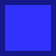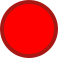#### You may also like### Jewellery Boxes

Can you find the value of the jewels?### Average Discovery

Find the missing number if the mean, median and mode are all the same.### Algebraic Average

The mean of three numbers x, y and z is x. What is the mean of y and z?

# Mean Balance

##### Age 14 to 16 ShortChallenge Level

Ifis less than 6, then adding anotherto the set would make the mean smaller.

Ifis more than 6, then adding anotherto the set would make the mean larger.

But adding anotherto the set does not change the mean, somust be exactly 6.

Alternatively:

If the mean of the seven numbers is 6, they must add up to 42.
If the mean of eight numbers is 6, they must add up to 48.
Therefore the eighth number is 48 - 42 = 6

So the mean of the set of numbers 8, 4, 5, 4,, 6, 6 is 6.

That means that ( 8 + 4 + 5 + 4 ++ 6 + 6 ) $\div$ 7 = 6,
so 8 + 4 + 5 + 4 ++ 6 + 6 = 42,
so 33 += 42, so= 9.

So= 9 and= 6.

You can find more short problems, arranged by curriculum topic, in our short problems collection.